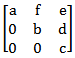Open in App
Not now

# GATE | GATE-CS-2005 | Question 46

• Last Updated : 09 Mar, 2018

Consider the set H of all 3 × 3 matrices of the typewhere a, b, c, d, e and f are real numbers and abc ≠ 0. Under the matrix multiplication operation, the set H is
(A) a group
(B) a monoid but not a group
(C) a semigroup but not a monoid
(D) neither a group nor a semigroup

Explanation: Because identity matrix is identity & as they define abc != 0, then it is non-singular so inverse is also defined.

The set of matrices is the set of Upper triangular matrices(H) of size 3*3 with non-zero determinant. Along with the multiplication operator the set forms an Algebraic Structure since it follows the Closure Property. This is because the product of Two Upper Triangular Matrices is also a Upper Triangular Matrix.
The Algebraic Structure also follows the Associative Property since, multiplication of matrices in general follows the Associative Property. Therefore it is a Semi Group.
The Algebraic Structure is also a Monoid, since it has an Identity element, which is the Identity Matrix- I3.
The Algebraic Structure is a Group since every matrix in H has an inverse, since every matrix in H is non-singular (given in question).
The Algebraic Structure is not an Abelian Group since it does not follow the Commutative Property.
Therefore Option A is correct.

This explanation is provided by Chirag Manwani.

Quiz of this Question

My Personal Notes arrow_drop_up